# l Integration Applications l Area Between Curves 6

• Slides: 9
Download presentation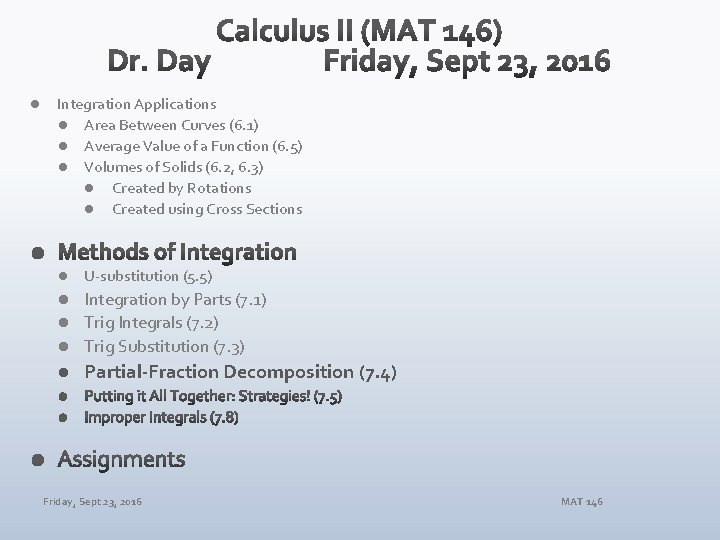l Integration Applications l Area Between Curves (6. 1) l Average Value of a Function (6. 5) l Volumes of Solids (6. 2, 6. 3) l Created by Rotations l Created using Cross Sections l U-substitution (5. 5) Integration by Parts (7. 1) l Trig Integrals (7. 2) l Trig Substitution (7. 3) l l Partial-Fraction Decomposition (7. 4) Friday, Sept 23, 2016 MAT 146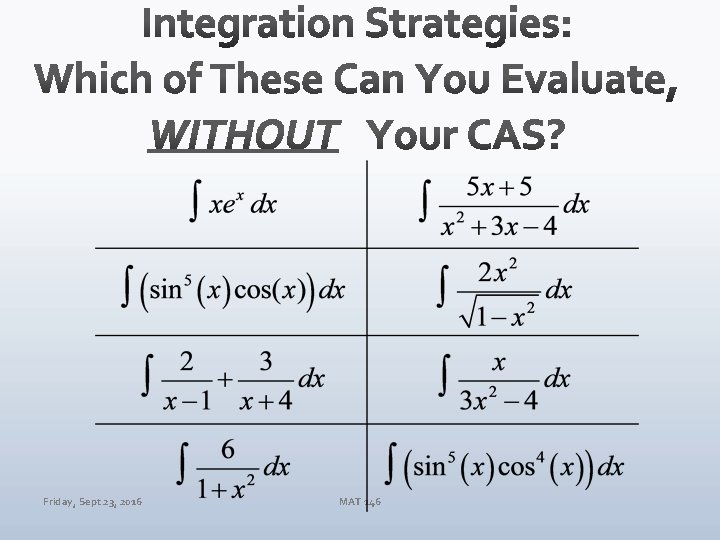Friday, Sept 23, 2016 MAT 146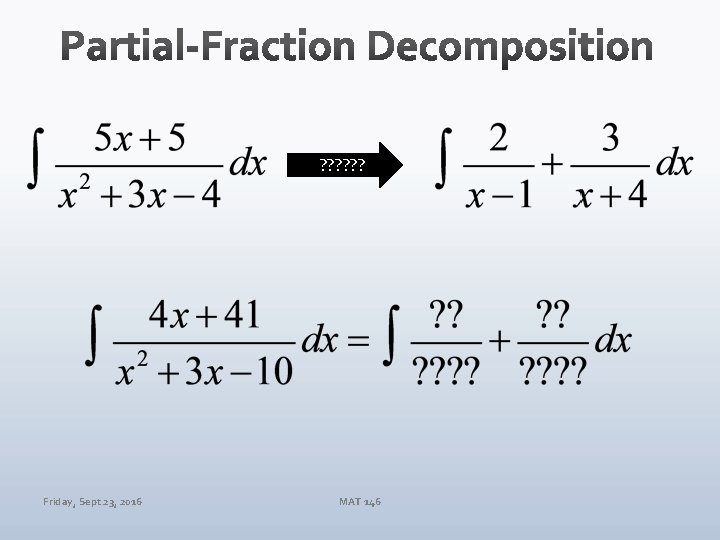? ? ? Friday, Sept 23, 2016 MAT 146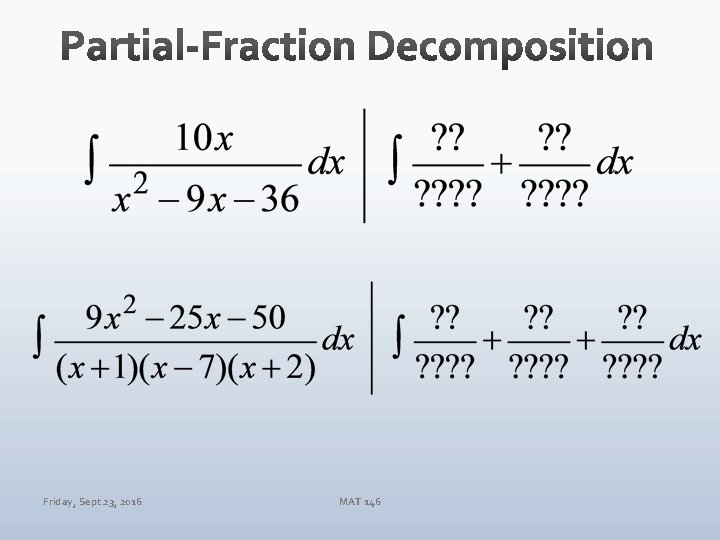Friday, Sept 23, 2016 MAT 146Improper Algebraic Fractions? Unfactorable Quadratics? Repeated Linear Factors? Friday, Sept 23, 2016 MAT 146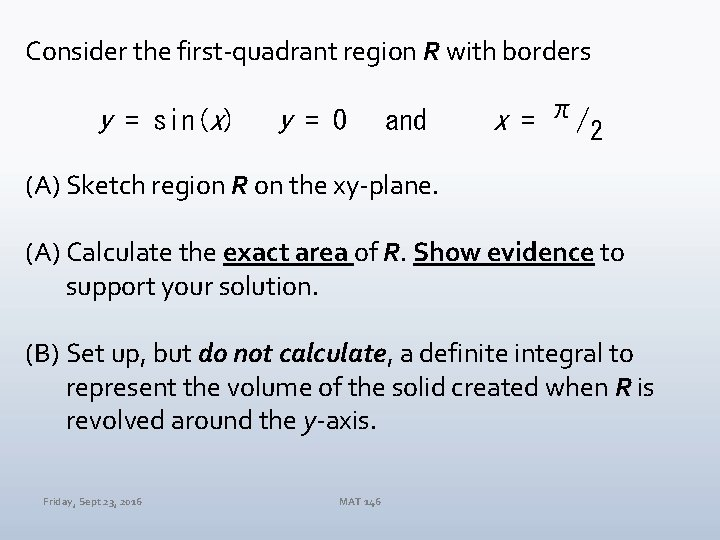Consider the first-quadrant region R with borders y = sin(x) y = 0 and x = π/2 (A) Sketch region R on the xy-plane. (A) Calculate the exact area of R. Show evidence to support your solution. (B) Set up, but do not calculate, a definite integral to represent the volume of the solid created when R is revolved around the y-axis. Friday, Sept 23, 2016 MAT 146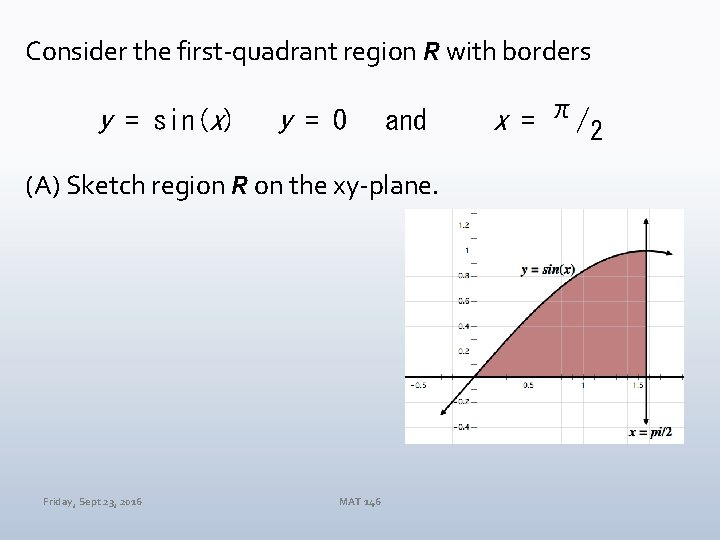Consider the first-quadrant region R with borders y = sin(x) y = 0 and (A) Sketch region R on the xy-plane. Friday, Sept 23, 2016 MAT 146 x = π/2Consider the first-quadrant region R with borders y = sin(x) y = 0 and x = π/2 B. Calculate the exact area of R. Show evidence to support your solution. Friday, Sept 23, 2016 MAT 146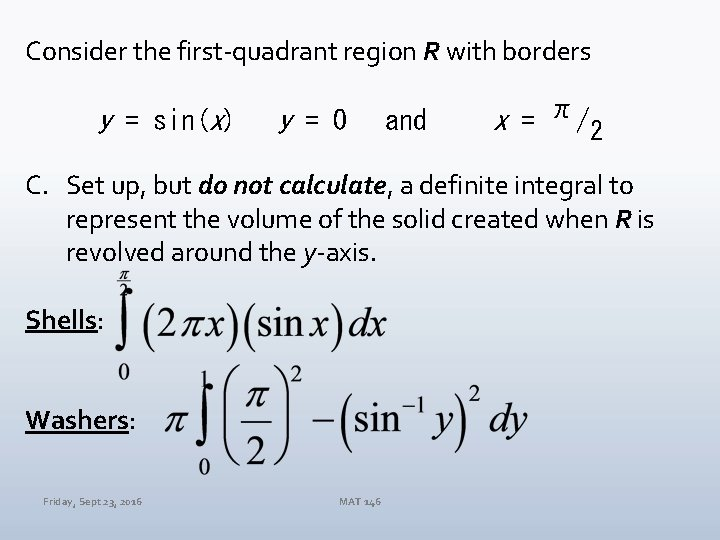Consider the first-quadrant region R with borders y = sin(x) y = 0 and x = π/2 C. Set up, but do not calculate, a definite integral to represent the volume of the solid created when R is revolved around the y-axis. Shells: Washers: Friday, Sept 23, 2016 MAT 146